## 90 Reputation

5 years, 212 days

## Explore with delayed evaluation?...

Maple

Hello everyone!

I am struggling to figure out the cause of the problem with Explore command. To put it as simple as possible:

a := <3, 4, 5>

Explore(a[i], i = 1 .. 3);
Error, (in Explore) bad index into Vector

I am pretty sure that this has to do with an attempt to evaluate A[i] with i not yet defined, but why does Explore behave this way? seq has no such problem:

seq(a[i], i = 1 .. 3);
3, 4, 5

But as soon as I put it into Explore, seq seems to forget all its "special evaluation rules":

Explore(seq(a[i], i = 1 .. 3), b = 1 .. 2);
Error, (in Explore) bad index into Vector

It is probably for the same reason that I can't solve the problem with unevaluation quotes such as:

Explore('a[i]', i = 1 .. 3);
Error, (in Explore) bad index into Vector

What am I doing wrong??

## "colorscheme" with "scaletorange" ?...

Maple 2018

Hello everyone!

In previous versions of Maple (e.g. Maple 2016) it used to be possible to use scaletorange and colorscheme options together as in:

densityplot(sin(x+y), x = -1 .. 1, y = -1 .. 1, colorscheme = [black, red, yellow, white], scaletorange = -.5 .. .5);

But Maple 2018 returns an error:

Error, (in plots/densityplot) the scaletorange option cannot be used with the colorscheme option

Why is that and can one work around this error in any simple way?

## What's wrong with the spherical version ...

Maple

How come I got this: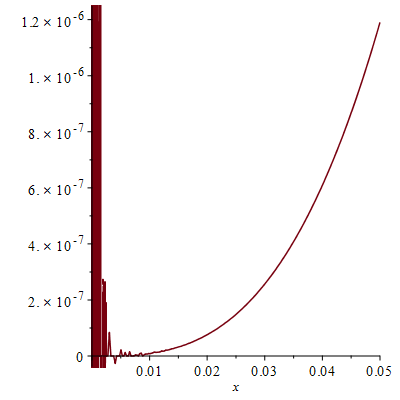as an output of plot(sqrt(Pi/(2*x))*BesselJ(3+1/2, x), x = 0 .. 0.5e-1),

and got this: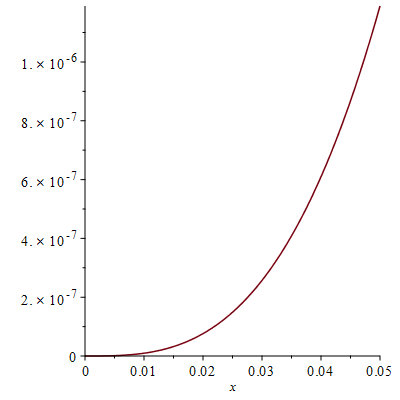for plot(sqrt(Pi/(2*x))*BesselJ(3+.5, x), x = 0 .. 0.5e-1).

Is 1/2 so much different from 0.5 to make Bessel function misbehave at small arguments?? Or is it just a bug?

Maple

Hello everyone!

I am confronted with a problem that seems to result from different definition of spherical coordinates in Maple and in Student[VectorCalculus] package.

I want to visualize a vector field defined in spherical coordinates (r,theta,phi).

A simple exampe is < 0, theta, 0 >. If I want to see just one slice of the field in OXY plane, I type:

fieldplot3d(<0, theta, 0>, r = 0 .. 1, theta = 0 .. 2*Pi, phi = 0 .. Pi, coords = spherical, grid = [10, 20, 3], color = black, fieldstrength = fixed(1), arrows = THICK)

And get: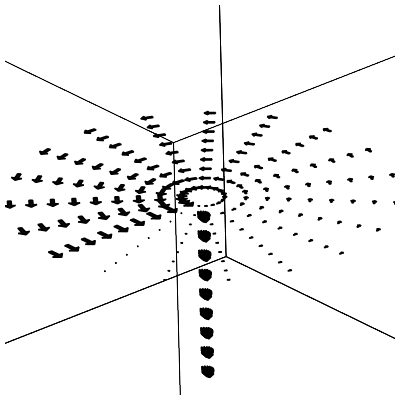which looks OK more or less but I don't like the nonuniform distribution of arrows. But since the field is defined in spherical coordinates there seems to be no way to produce a uniform distribution with fieldplot3d command.

However VectorField command in Student[VectorCalculus] package seems to do just what I want. But a similar call to it:

SetCoordinates('spherical'[r, theta, phi])

VectorField(<0, theta, 0>, output = plot, view = [-5 .. 5, -5 .. 5, -10 .. 10], fieldoptions = [arrows = THICK, color = black, grid = [10, 10, 3], view = [-5 .. 5, -5 .. 5, -1 .. 1]])  produces quite a different result: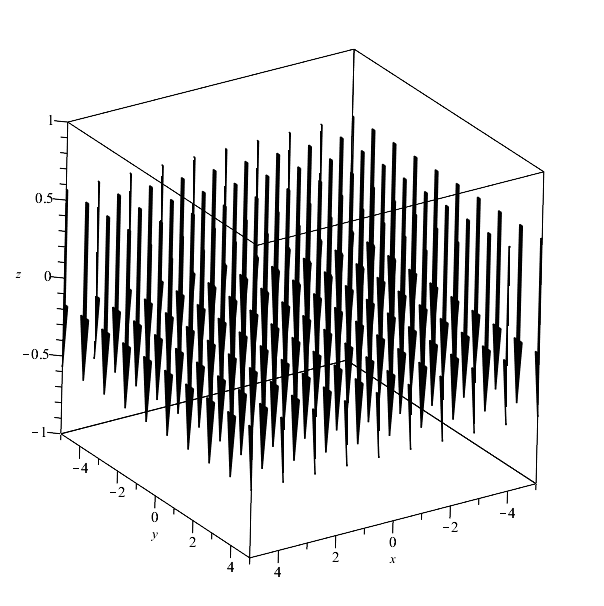which means that the second argument 'theta' is not the azimuthal angle but the angle from the Z axis, and amounts to Pi/2 since we look at the OXY plane.

The result of: VectorField(<0, 0, theta>, output = plot, view = [-5 .. 5, -5 .. 5, -10 .. 10], fieldoptions = [arrows = THICK, color = black, grid = [10, 10, 3], view = [-5 .. 5, -5 .. 5, -1 .. 1]])  looks better: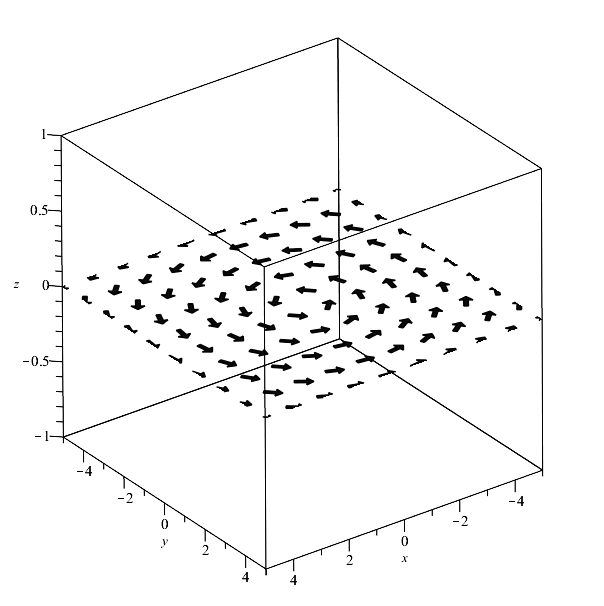but is not correct since the magnitude of the field does not change with the azimutal angle. Finally,

VectorField(<0, 0, phi>, output = plot, view = [-5 .. 5, -5 .. 5, -10 .. 10], fieldoptions = [arrows = THICK, color = black, grid = [10, 10, 3], view = [-5 .. 5, -5 .. 5, -1 .. 1]]) produces almost what I want: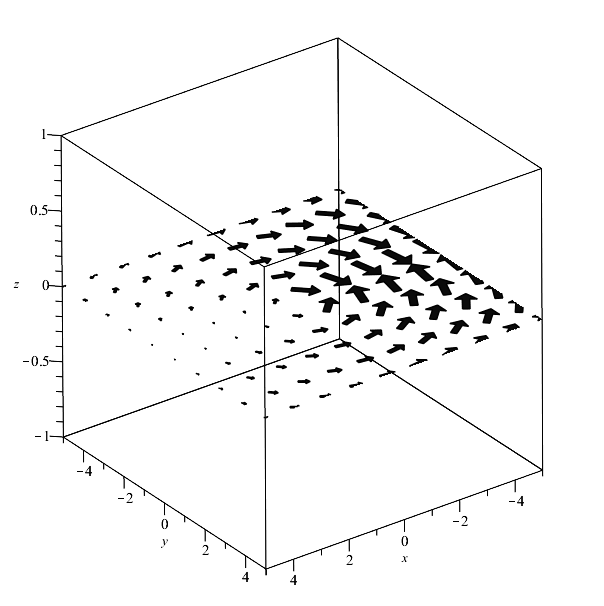but it's quite different from what you get with fieldplot3d command. Apparently, not only azimuthal angle is now the third argument but it varies from -Pi to Pi, not from 0 to 2Pi as it does in fieldplot3d. I hoped to use both fieldplot3d and VectorField  with my vector fields but I cannot since they are not consistent in the definition of spherical coordinates.

My questions therefore are:

1) Is there a way to redifine spherical coordinates in VectorField command to make them consistent with the rest of Maple?

2) Can I produce a uniform 3D distribution of arrows for a field in spherical coordinates without VectorField command?

## How to color a 3D surface based on the m...

Maple

Hello everyone!

I have a vector field defined in spherical coordinates and I want to plot a sphere colored according to the magnitude of the vector field on that sphere. Is there a simple way to do this?

 1 2Page 1 of 2